# Algorithm Design and Analysis: Branch and Bound

Directory

Level 1: 0/1 knapsack problem

mission details:

related information:

Basic idea of branch and bound method:

Common branch and bound methods:

Example: 0/1 knapsack problem

For 0/1 knapsack optimization:

Title description:

Programming requirements:

Test instruction:

Level 2: The Traveling Salesman Problem

mission details:

related information:

Problem Description:

problem analysis:

Programming requirements:

Test instruction:

## Level 1: 0/1 knapsack problem

The task of this level: branch and limit method;

In order to complete the task of this level, you need to master: 0/1 backpack problem;

### Basic idea of branch and bound method:

The branch and bound method is a classic method for solving pure integer programming or mixed integer programming problems. It was proposed by Land Doig and Dakin et al. in the 1960s. This method is flexible and easy to solve by computer. It has been successfully used to solve production schedule problems, traveling salesman problems, factory location problems, knapsack problems and distribution problems. The basic idea of the algorithm is as follows:

1. Search the problem’s solution space tree in a breadth-first or minimum-cost (maximum-efficiency)-first manner.
2. In the branch and bound method, each live node has only one chance to become an expansion node. Once a live node becomes an expansion node, all its child nodes will be generated at one time, and the child nodes that lead to infeasible solutions or non-optimal solutions is discarded, and the remaining son nodes are added to the live node table.
3. Then take the next node from the live node table to become the current extended node.
4. Repeat the above node expansion process until the required solution is found or the live node list is empty.

### Common branch and bound methods:

Queue branch and bound method Select the next node as the expansion node according to the principle of first-in-first-out (FIFO) Point tables have the same properties as queues

Priority queue branch and bound method (minimum cost or maximum benefit) Each node has a corresponding cost or benefit, so as to determine the priority of the node Select the node with the highest priority from the priority queue The node becomes the current expansion node. If you want to search for a solution with the minimum cost: the live node table can be built with a small top heap, and the next expansion node is the live node with the minimum cost. If you want to search for a solution with the maximum benefit: you can use it The big top heap is used to construct the live node table, and the next expanded node is the live node with the maximum benefit

### Example: 0/1 knapsack problem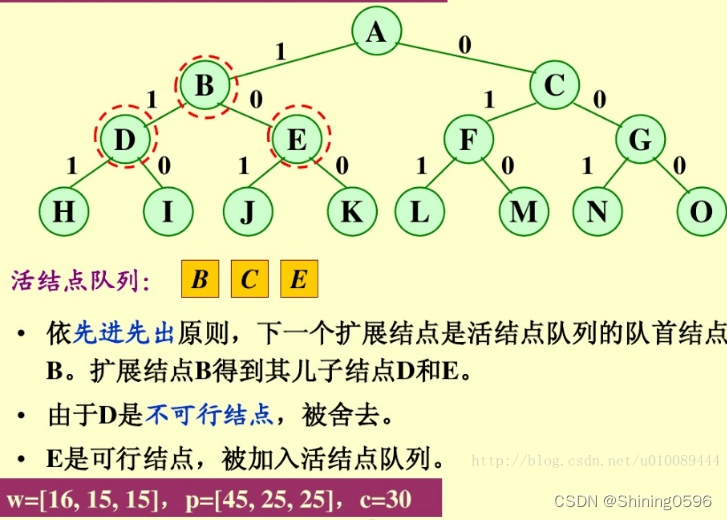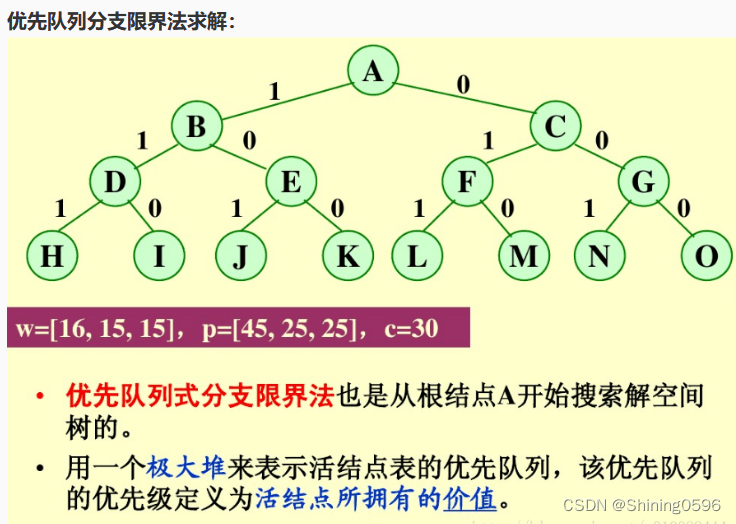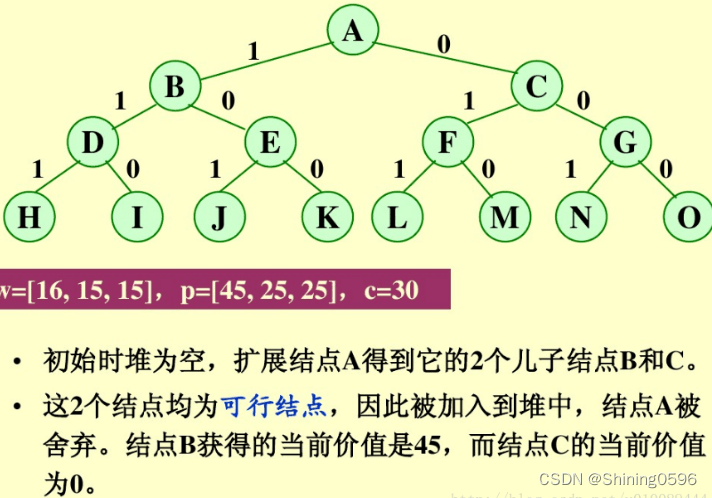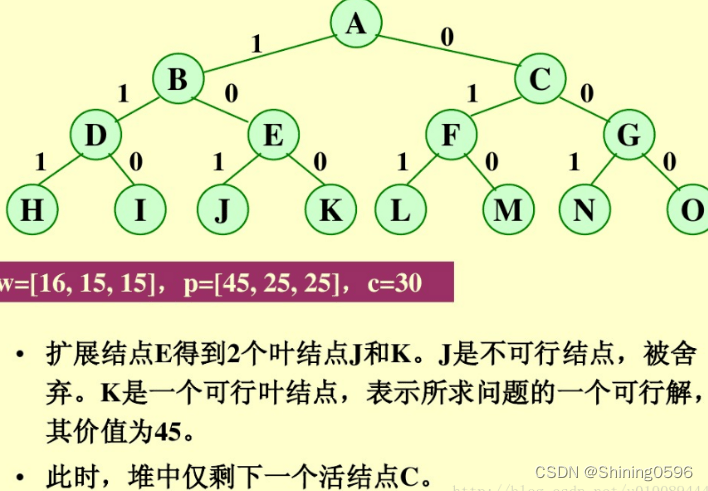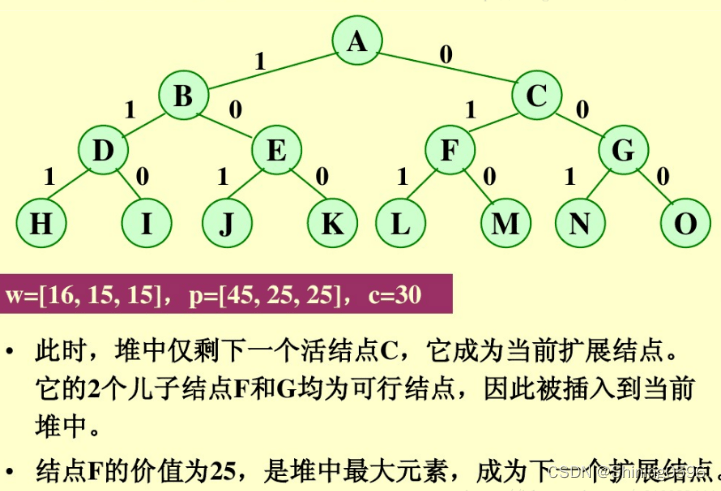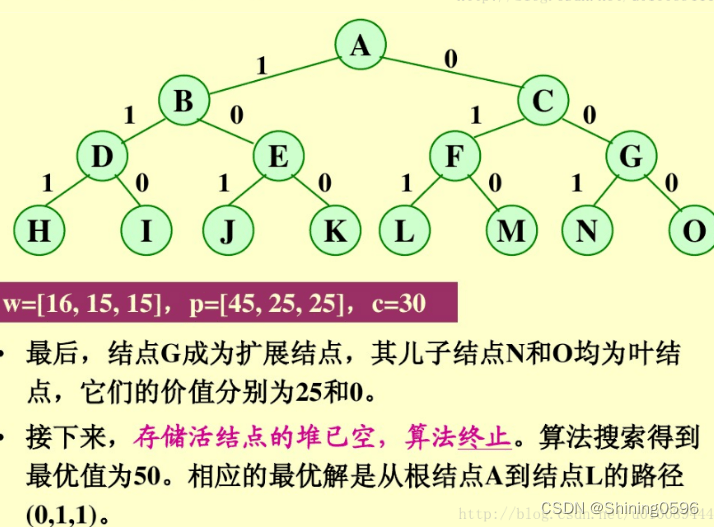### Optimization for 0/1 backpack:

First of all, it is necessary to preprocess the input data, and arrange the items according to their unit weight value from large to small. In the branch-and-bound method of the priority queue, the priority of the node is the sum of the value of the bagged item plus the value of the remaining capacity of the item with the largest unit weight value. The algorithm first checks the feasibility of the left child node of the currently expanded node. If the left child node is a feasible node, it will be added to the subset tree and live node priority queue. The right child node of the current expanded node must be a feasible node, and only when the right child node satisfies the upper bound constraint will it be added to the subset tree and the active node priority queue. It is the optimal value of the problem when extended to the leaf nodes.

### Description of topic:

0-1 Knapsack Problem: Given n items and a knapsack. The weight of item i is wi, its value is Vi, and the capacity of the knapsack is C. How to choose the items in the knapsack so that the total value of the items in the knapsack is the largest? When selecting the items in the knapsack, you can choose a part of this item for each item i.

## Programming Requirements:

According to the prompt, supplement the code in the editor on the right, and traverse the hierarchy of the tree.

Input: read in through the file, the first line includes two integers n and c, representing the number of items and the capacity of the backpack, and each of the next n lines includes two integers, \$w[i]\$ and \$v[i]\$ Represents the weight and value of an item. Output: Output the calculation results to the file, including the optimal value and the selection plan, including 2 lines, the first line is an integer, indicating the calculation result, and the second line includes a set of sequences, indicating the selection plan, according to \$T={1,0 , 1, 1}\$, expressed in matrix form. Requires a branch and bound solution.

## Test Description:

Test input:

1. `10 34`
2. `2 15`
3. `8 25`
4. `4 9`
5. `4 9`
6. `8 15`
7. `7 12`
8. `8 12`
9. `5 6`
10. `16 14`
11. `16 9`

Expected output:

1. `85`
```#include <iostream>
using namespace std;

int maxSize=0;
/**********Begin**********/
void backtrack(int * weights, int * values, int sum, int value, int totalCapacity, int index, int num){

if(value >maxSize){
maxSize = value;
}
if (index>=num) {
return;
}
for (int i = 0; i <= 1; i ++ ) {
if(sum + weights[index]*i <= totalCapacity){
backtrack(weights, values, sum + weights[index] * i, value + values[index] * i, totalCapacity, index + 1, num);
}
}
}
/**********End ***********/

int main() {
/**********Begin**********/
int n;
int capacity;

cin >> n;
cin >> capacity;
int* weights = new int[n];
int* values = new int[n];

for (int i = 0; i < n; i ++ ) {
cin >> weights[i];
cin >> values[i];
}
backtrack(weights, values, 0, 0, capacity, 0, n);
cout << maxSize << endl;
delete[] weights;
delete[] values;
/**********Begin**********/
return 0;
}
```

## Level 2: Traveling salesman problem

The task of this level: the traveling salesman problem.

## Related knowledge:

In order to complete the task of this level, you need to master: branch and limit method.

### Problem Description:

A salesman wants to go to several cities to sell goods, and the distance between the cities is known. He wants to choose a route starting from the station, passing through each city, and finally returning to the place of residence, so that the total distance is the shortest.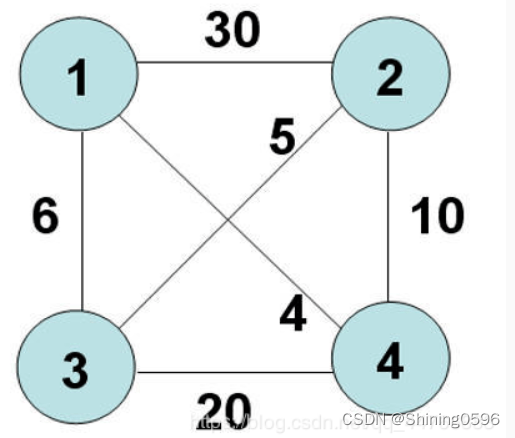The result is: `1 3 2 4 1`

### Problem Analysis:

Priority Queue Branch-and-Bound Method to Solve Traveling Salesman Problem `Using priority queue to store live node table` The `priority definition` of live node m in priority queue is: `live node The subtree cost lower bound lcost` corresponding to m. `lcost=cc + rcost`, where cc is the cost of the current node, and rcost is the sum of `the minimum outgoing cost of the current vertex plus the minimum outgoing cost of all remaining vertices`. The active node with the `highest priority` in the priority queue becomes the next expanded node. The load capacity corresponding to the leaf node in the arrangement tree is the same as its priority (lower limit value), that is: `The cost (bestc) of the circuit corresponding to the leaf node is equal to the value of the lower bound lcost of the subtree cost`. Similar to the discussion of subset trees, there are two different implementations of the `priority-queue branch-and-bound method` for permutation tree search: (Traveling salesman problem adopts `first implementation `.)

1. Use a priority queue to store live nodes. `Each active node in the priority queue stores the corresponding path` from the root to the active node.
2. Use the priority queue to store live nodes, and at the same time store the `currently constructed partial permutation tree`. A live node in a priority queue does not have to store the corresponding path from the root to the live node, which path is `obtained` from the stored partial permutation tree if necessary.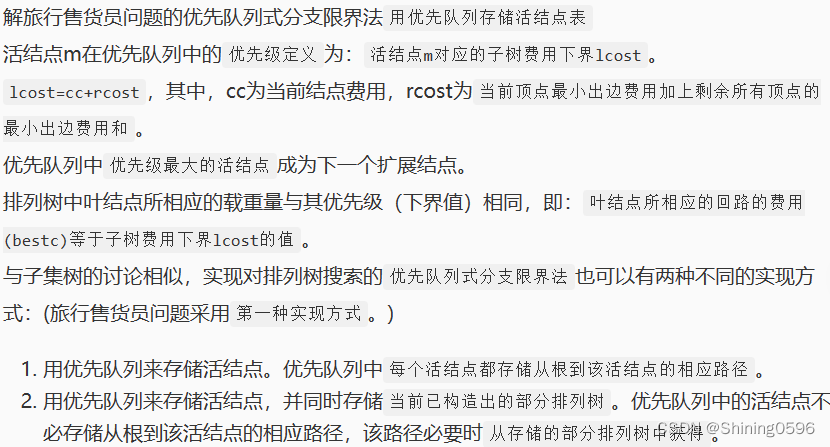## Programming Requirements:

According to the prompt, supplement the code in the editor on the right to calculate and output the average value and maximum value of the array.

## Test Description:

Input data: In the first line, there are n cities. The next n rows and n columns represent the direct distance between n cities. If there is no connection, use -1 instead. Output data: one row. The optimal value, the optimal solution The platform will test the code you write:

The platform will test the code you write:

Test input:

1. `4`
2. `-1 30 6 4`
3. `30 -1 5 10`
4. `6 5 -1 20`
5. `4 10 20 -1`

Expected output:

1. `The optimal value is: 25 The optimal solution is: 1423`
```#include <iostream>
#define NO_PATH -1 //No path
#define MAX_WEIGHT 4000

using namespace std;
/**********Begin**********/
// functions, global variables, structures, etc.
int N; //Number of cities
int City_Graph; //Save graph information
int x; //x[i] saves the city traversed in step i
int isIn; //Save whether city i has been added to the path
int bestw; //The total weight of the optimal path
int cw; // total weight of the current path
int bestx; //optimal path
void Travel_Backtrack(int t){ //recursive method
int i,j;
if(t>N){ // finished, output the result
if(cw < bestw){ //Judge whether the current path is a better solution
for (i=1;i<=N;i + + ){
bestx[i] = x[i];
}
bestw = cw + City_Graph[x[N]];
}
return;
}
else {
for(j=1;j<=N;j + + ){ //Find the city that can be walked in step t
if(City_Graph[x[t-1]][j] != NO_PATH & amp; & amp; !isIn[j]){ //Able to arrive but not added to the path
isIn[j] = 1;
x[t] = j;
cw + = City_Graph[x[t-1]][j];
Travel_Backtrack(t + 1);
isIn[j] = 0;
x[t] = 0;
cw -= City_Graph[x[t-1]][j];
}
}
}
}
/**********End ***********/

int main(){
/**********Begin**********/
int i;
cin>>N;
for(int i=1;i<=N;i + + ){

for(int j=1;j<=N;j++){
cin>>City_Graph[i][j];
}
}

// test recursion
for (i=1;i<=N;i + + ){
x[i] = 0; //Indicates that step i has no solution yet
bestx[i] = 0; //There is no optimal solution yet
isIn[i] = 0; //Indicates that the i-th city has not been added to the path
}

x = 1; //The first step is to go to city 1
isIn = 1; //Add the first city to the path
bestw = MAX_WEIGHT;
cw = 0;
Travel_Backtrack(2); //Choose the city from the second step
cout<<"The optimal value is:"<<bestw;
cout<<"The optimal solution is:";
for(i=1;i<=N;i + + ){
cout<<bestx[i];
}
cout<<endl;
/**********End ***********/
return 0;
}
```